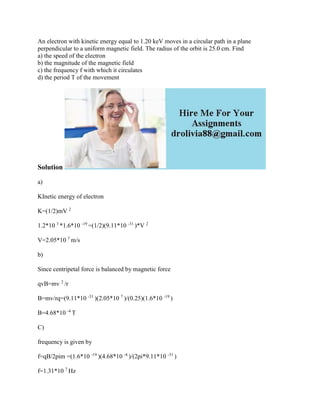Successfully reported this slideshow.

# An electron with kinetic energy equal to 1-20 keV moves in a circular.docx×

# An electron with kinetic energy equal to 1-20 keV moves in a circular.docx

An electron with kinetic energy equal to 1.20 keV moves in a circular path in a plane perpendicular to a uniform magnetic field. The radius of the orbit is 25.0 cm. Find
a) the speed of the electron
b) the magnitude of the magnetic field
c) the frequency f with which it circulates
d) the period T of the movement
Solution
a)
KInetic energy of electron
K=(1/2)mV 2
1.2*10 3 *1.6*10 -19 =(1/2)(9.11*10 -31 )*V 2
V=2.05*10 7 m/s
b)
Since centripetal force is balanced by magnetic force
qvB=mv 2 /r
B=mv/rq=(9.11*10 -31 )(2.05*10 7 )/(0.25)(1.6*10 -19 )
B=4.68*10 -4 T
C)
frequency is given by
f=qB/2pim =(1.6*10 -19 )(4.68*10 -4 )/(2pi*9.11*10 -31 )
f=1.31*10 7 Hz
d)
Period
T=1/f =7.66*10 -8 s
.

An electron with kinetic energy equal to 1.20 keV moves in a circular path in a plane perpendicular to a uniform magnetic field. The radius of the orbit is 25.0 cm. Find
a) the speed of the electron
b) the magnitude of the magnetic field
c) the frequency f with which it circulates
d) the period T of the movement
Solution
a)
KInetic energy of electron
K=(1/2)mV 2
1.2*10 3 *1.6*10 -19 =(1/2)(9.11*10 -31 )*V 2
V=2.05*10 7 m/s
b)
Since centripetal force is balanced by magnetic force
qvB=mv 2 /r
B=mv/rq=(9.11*10 -31 )(2.05*10 7 )/(0.25)(1.6*10 -19 )
B=4.68*10 -4 T
C)
frequency is given by
f=qB/2pim =(1.6*10 -19 )(4.68*10 -4 )/(2pi*9.11*10 -31 )
f=1.31*10 7 Hz
d)
Period
T=1/f =7.66*10 -8 s
.